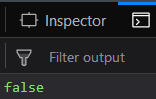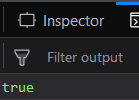# Underscore.js _.isFinite() Function

• Last Updated : 25 Nov, 2021

The _.isFinite() function is used to check whether the value of the parameter passed is finite or not. If the parameter has an infinite value the output is false otherwise, true. We can even perform any operation like addition, subtraction in this function.

Syntax:

`_.isFinite(object)`

Parameters:
It takes only one argument which is the argument that needs to be checked.

Return value:
It returns true if the parameter has a finite value otherwise it returns false.

Examples:

1. Passing a positive number to the _.isFinite() function:
The _.isFinite() function takes the number that is passed to it. Since every number have a finite value, therefore when it will check it will declare it’s parameter passed as a finite variable. And hence the output will be false.

## html

 ```<``html``>``   ` `<``head``>``    ``<``script` `src` `= ``"https://cdnjs.cloudflare.com/ajax/libs/underscore.js/1.9.1/underscore-min.js"` `>``    ``````   ` `<``body``>``    ``<``script` `type``=``"text/javascript"``>``        ``console.log(_.isFinite(10));``    ``````   ` ``

Output:2. Passing a negative number to the _.isFinite() function:
The _.isFinite() function takes the number that is passed to it. Since, it is a negative number but still have a value so, for the same reason as above the _.isFinite() function will declare it’s parameter passed as a finite variable. And hence the output will be false.

## html

 ```<``html``>``   ` `<``head``>``    ``<``script` `src` `= ``"https://cdnjs.cloudflare.com/ajax/libs/underscore.js/1.9.1/underscore-min.js"` `>``    ``````   ` `<``body``>``    ``<``script` `type``=``"text/javascript"``>``        ``console.log(_.isFinite(-10));``    ``````   ` ``

Output:3. Passing a defined variable to the _.isFinite() function:
The _.isFinite() function takes the parameter passed to it which is variable ‘a’ here. Then it checks the value of ‘a’ which is 10. It is not finite. Therefore, the answer is false.

## html

 ```<``html``>``   ` `<``head``>``    ``<``script` `src` `= ``"https://cdnjs.cloudflare.com/ajax/libs/underscore.js/1.9.1/underscore-min.js"` `>``    ``````   ` `<``body``>``    ``<``script` `type``=``"text/javascript"``>``        ``var a=1000;``        ``console.log(_.isFinite(a));``    ``````   ` ``

Output:4. Passing a variable without initializing to the _.isFinite() function:
The _.isFinite() function takes the parameter passed to it which is variable ‘a’ here. Then it checks the value of ‘a’ which is not defined and hence is not fixed. It means ‘a’ has infinite values. Therefore, the answer is true.

## html

 ```<``html``>``   ` `<``head``>``    ``<``script` `src` `= ``"https://cdnjs.cloudflare.com/ajax/libs/underscore.js/1.9.1/underscore-min.js"` `>``   ``````   ` `<``body``>``    ``<``script` `type``=``"text/javascript"``>``        ``var a;``        ``console.log(_.isFinite(a));``    ``````   ` ``

Output:NOTE: These commands will not work in Google console or in firefox as for these additional files need to be added which they didn’t have added.
 ```<``script` `type``=``"text/javascript"` `src` `=``"https://cdnjs.cloudflare.com/ajax/libs/underscore.js/1.9.1/underscore-min.js"``>```